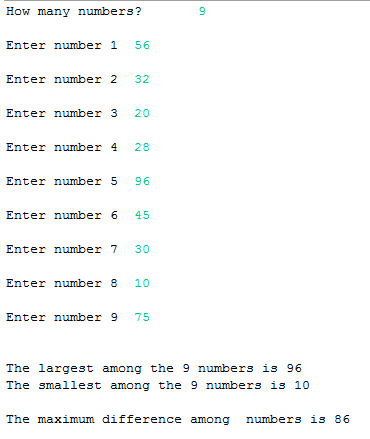## Saturday, 10 January 2015

### Java Program to find the maximum difference between all the elements in an array

This is a Java Program which finds out the maximum difference between the elements in an array.

Here first we find the largest element in the array and store the result and then find the smallest element in the array and store the result .
Now substract the largest element with the smallest element , this gives us the maximum difference in an array.

PROGRAM :
```package codingcorner.in;

import java.util.Scanner;

public class MaxDiffArray {
public static void main(String[] args) {
int i, n, l, s;
Scanner scan = new Scanner(System.in);
System.out.print("How many numbers? \t");
n = scan.nextInt();
int array[] = new int[n];
int dup[] = new int[n];
for (i = 0; i < n; i++) {
System.out.print("\nEnter number " + (i + 1) + " \t");
array[i] = scan.nextInt();
dup[i] = array[i]; // storing the copy of of array[]
}
scan.close();

for (i = 0; i < n; i++) // checking for largest
{
if (array < array[i])
array = array[i];
}
l = array; // storing largest num
System.out.print("\n\nThe largest among the " + n + " numbers is "+ array);

for (i = 0; i < n; i++) // checking for smallest
{
if (dup > dup[i]) // we used duplicate because original array[] has changed
dup = dup[i];
}
s = dup; // storing smallest
System.out.print("\nThe smallest among the " + n + " numbers is "+ dup);
System.out.print("\n\nThe maximum difference among  numbers is "+ (l - s));
}
}
```

OUTPUT :Java - Maximum difference between all the elements in an array